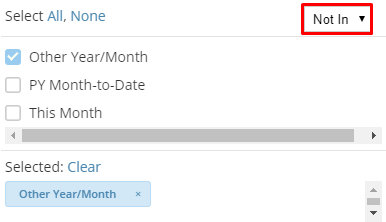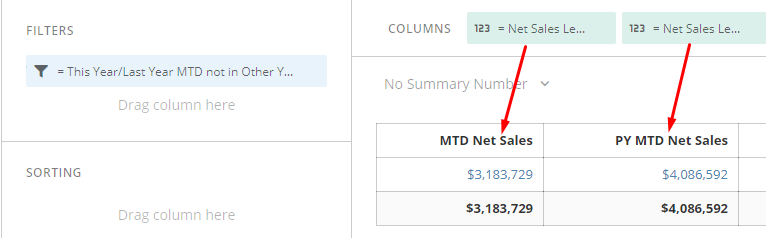# Beast Mode Period-over-Period issues

Okay, so I'm trying to do 4 Beast Modes, and I cannot seem to get the right data.  I'm trying to find the average amount of dollars per time period.  Here's what I have come up with so far, to no avail:

• Current year-to-date
• avg(CASE WHEN `Date` >= DATE_FORMAT(CURDATE(), '%Y-01-01')
THEN `Dollars column` END)  or
• AVG(CASE WHEN `Date` <= DATE_SUB(CURDATE(),INTERVAL 0 YEAR) AND `Date` >= DATE_FORMAT(DATE_SUB(CURDATE(),INTERVAL 0 YEAR), '%Y-01-01')
THEN `Dollars column` END)
• Previous year-to-date
• AVG(CASE WHEN `Date` <= DATE_SUB(CURDATE(),INTERVAL 1 YEAR) AND `Date` >= DATE_FORMAT(DATE_SUB(CURDATE(),INTERVAL 1 YEAR), '%Y-01-01')
THEN `Dollars column` END)
• Current year, current month
• avg(CASE WHEN `Date` >= DATE_FORMAT(CURDATE(MONTH()), '%Y-01-01')
THEN `Dollars column` END)
• Previous year, current month
• AVG(CASE WHEN `Date` <= DATE_SUB(CURDATE(MONTH()),INTERVAL 1 YEAR) AND `Date` >= DATE_FORMAT(DATE_SUB(CURDATE(MONTH()),INTERVAL 1 YEAR), '%Y-01-01')
THEN `Dollars column` END)

What am I missing?  The only column I'm getting any data out of is Current year-to-date, and the Current year/Current month is giving me the same data.

Greggory S. Wright, PhD

• I can give you a two card approach that will allow you to build a Table that displays MTD Sales next to PY MTD sales on one card and then YTD Sales next to PY YTD Sales.

First, we are going to create a "Filter Series" that we will drop in the Filters section of the card. The reason for this is that we are going to build the card with a default timeframe of "All Time" and then limit the card to only data from this year and last year:

`/* This Year/Last Year MTD  */CASE WHEN YEAR(`happened_at`) = YEAR(CURRENT_DATE()) AND MONTH(`happened_at`) = MONTH(CURRENT_DATE()) THEN 'This Month'      WHEN YEAR(`happened_at`)= (YEAR(CURRENT_DATE()) - 1)      	AND MONTH(`happened_at`) = MONTH(CURRENT_DATE())         AND DAYOFMONTH(`happened_at`) <= (DAYOFMONTH(DATE(CURRENT_DATE()))-1) THEN 'PY Month-to-Date'     ELSE 'Other Year/Month' END/*Read PY Month-to-Date as: When year = last year AND the month number is the same as the current month's number AND the day of the month is less than or equal to yesterday (today - 1) */`Now we will create two new Measures by writing a beast mode for This Year's sales data:

`IFNULL(SUM(CASE WHEN YEAR(CURRENT_DATE()) = YEAR(`happened_at`) THEN `sales_data` end),0)`

and Last Year's sales data:

`IFNULL(SUM(CASE WHEN YEAR(CURRENT_DATE())-1 = YEAR(`happened_at`) THEN `sales_data` end),0)`

Now, when you drag those two Measures in to a Table card in separate columns you should see the respective numbers. You can replace SUM with AVG if that fits your needs better.

Now for Year to Date...

Same thing where we want to first create a filter for our data to limit it to only YTD and PY YTD data:

`CASE WHEN YEAR(`happened_at`) = YEAR(CURRENT_DATE())  THEN 'This Year'      WHEN YEAR(`happened_at`) = (YEAR(CURRENT_DATE()) - 1) AND DAYOFYEAR(CURRENT_DATE()) >= (DAYOFYEAR(`happened_at`)+1) THEN 'Last Year'     ELSE 'Other Year' END/* Read 'Last Year' as: when Year = Last Year AND Dayofyear is less than current date - 1 (yesterday) ... NOTE: There is some sort of "bug" with the DAYOFYEAR function in which you have to use the inverse logic, so while I wrote "...greater than or equal to the current date + 1"  the result is that it yields "less than or equal to yesterday's date */`

Drop that in the Filters section of the Analyzer and choose Not In 'Other Year.' Then we can actually use the exact same Measures that we crated before for This Year's sales data and Last Years sales data (see the one line beast modes above).

For your MTD vs PY MTD your card should look something like this:I hope that at least helps you get closer with a theortecial approach as well as a good bit of beast mode syntax that you can break down/experiment with.

• I can give you a two card approach that will allow you to build a Table that displays MTD Sales next to PY MTD sales on one card and then YTD Sales next to PY YTD Sales.

First, we are going to create a "Filter Series" that we will drop in the Filters section of the card. The reason for this is that we are going to build the card with a default timeframe of "All Time" and then limit the card to only data from this year and last year:

`/* This Year/Last Year MTD  */CASE WHEN YEAR(`happened_at`) = YEAR(CURRENT_DATE()) AND MONTH(`happened_at`) = MONTH(CURRENT_DATE()) THEN 'This Month'      WHEN YEAR(`happened_at`)= (YEAR(CURRENT_DATE()) - 1)      	AND MONTH(`happened_at`) = MONTH(CURRENT_DATE())         AND DAYOFMONTH(`happened_at`) <= (DAYOFMONTH(DATE(CURRENT_DATE()))-1) THEN 'PY Month-to-Date'     ELSE 'Other Year/Month' END/*Read PY Month-to-Date as: When year = last year AND the month number is the same as the current month's number AND the day of the month is less than or equal to yesterday (today - 1) */`Now we will create two new Measures by writing a beast mode for This Year's sales data:

`IFNULL(SUM(CASE WHEN YEAR(CURRENT_DATE()) = YEAR(`happened_at`) THEN `sales_data` end),0)`

and Last Year's sales data:

`IFNULL(SUM(CASE WHEN YEAR(CURRENT_DATE())-1 = YEAR(`happened_at`) THEN `sales_data` end),0)`

Now, when you drag those two Measures in to a Table card in separate columns you should see the respective numbers. You can replace SUM with AVG if that fits your needs better.

Now for Year to Date...

Same thing where we want to first create a filter for our data to limit it to only YTD and PY YTD data:

`CASE WHEN YEAR(`happened_at`) = YEAR(CURRENT_DATE())  THEN 'This Year'      WHEN YEAR(`happened_at`) = (YEAR(CURRENT_DATE()) - 1) AND DAYOFYEAR(CURRENT_DATE()) >= (DAYOFYEAR(`happened_at`)+1) THEN 'Last Year'     ELSE 'Other Year' END/* Read 'Last Year' as: when Year = Last Year AND Dayofyear is less than current date - 1 (yesterday) ... NOTE: There is some sort of "bug" with the DAYOFYEAR function in which you have to use the inverse logic, so while I wrote "...greater than or equal to the current date + 1"  the result is that it yields "less than or equal to yesterday's date */`

Drop that in the Filters section of the Analyzer and choose Not In 'Other Year.' Then we can actually use the exact same Measures that we crated before for This Year's sales data and Last Years sales data (see the one line beast modes above).

For your MTD vs PY MTD your card should look something like this:I hope that at least helps you get closer with a theortecial approach as well as a good bit of beast mode syntax that you can break down/experiment with.

• Thank you so much @DDalt

Greggory S. Wright, PhD